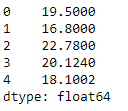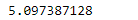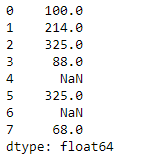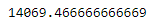Related Articles

# Python | Pandas Series.var

• Last Updated : 28 Jan, 2019

Python is a great language for doing data analysis, primarily because of the fantastic ecosystem of data-centric python packages. Pandas is one of those packages and makes importing and analyzing data much easier.

Pandas series is a One-dimensional ndarray with axis labels. The labels need not be unique but must be a hashable type. The object supports both integer- and label-based indexing and provides a host of methods for performing operations involving the index.

Pandas` Series.var()` function return unbiased variance over requested axis. The variance is normalized by N-1 by default. This can be changed using the ddof argument.

Syntax: Series.var(axis=None, skipna=None, level=None, ddof=1, numeric_only=None, **kwargs)

Parameter :
axis : {index (0)}
skipna : Exclude NA/null values. If an entire row/column is NA, the result will be NA
level : If the axis is a MultiIndex (hierarchical), count along a particular level, collapsing into a scalar
ddof : Delta Degrees of Freedom. The divisor used in calculations is N – ddof, where N represents the number of elements.
numeric_only : Include only float, int, boolean columns. If None, will attempt to use everything, then use only numeric data. Not implemented for Series.

Returns : var : scalar or Series (if level specified)

Example #1: Use `Series.var()` function to find the variance of the given Series object.

 `# importing pandas as pd``import` `pandas as pd`` ` `# Creating the Series``sr ``=` `pd.Series([``19.5``, ``16.8``, ``22.78``, ``20.124``, ``18.1002``])`` ` `# Print the series``print``(sr)`

Output :Now we will use `Series.var()` function to find the variance of the given series object.

 `# find the variance``sr.var()`

Output :As we can see in the output, the `Series.var()` function has returned the variance of the given Series object.

Example #2: Use `Series.var()` function to find the variance of the given Series object. The given Series object contains some missing values.

Note : We can skip the missing values by setting the skipna parameter to `True`.

 `# importing pandas as pd``import` `pandas as pd`` ` `# Creating the Series``sr ``=` `pd.Series([``100``, ``214``, ``325``, ``88``, ``None``, ``325``, ``None``, ``68``])`` ` `# Print the series``print``(sr)`

Output :Now we will use `Series.var()` function to find the variance of the given series object.

 `# find the variance``sr.var(skipna ``=` `True``)`

Output :As we can see in the output, the `Series.var()` function has returned the variance of the given Series object.

Attention geek! Strengthen your foundations with the Python Programming Foundation Course and learn the basics.

To begin with, your interview preparations Enhance your Data Structures concepts with the Python DS Course. And to begin with your Machine Learning Journey, join the Machine Learning – Basic Level Course

My Personal Notes arrow_drop_up